# Trigonometric Equations

GCSELevel 8-9Cambridge iGCSE

## Solving Trigonometric Equations

Using what we know already about sine, cosine, and tangent, (or sin, cos, and tan), we need to be able to solve equations involving these.

For example,

$\sin(x)=\dfrac{\sqrt{3}}{2}$

We will look at how to solve equations like this graphically, and algebraically

## Solving Graphically

We can look at the sin, cos, and tan graphs to solve equations.

For example, estimate the solutions to

$\sin(x)=0.2$

$\cos(x)=0.2$

$\tan(x)=0.2$

Between $0\degree$ and $360\degree$

Sine graph

To solve, draw a line on the sine graph at $y=0.2$ and read off the solutions: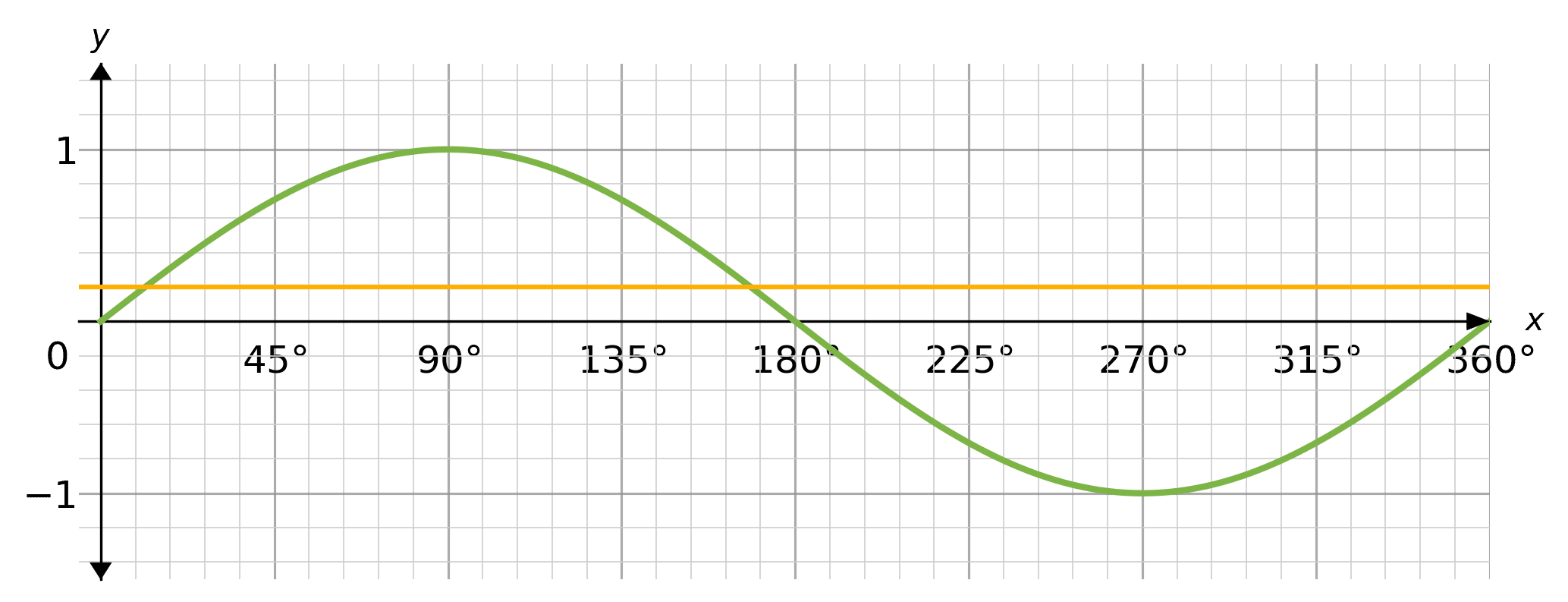The yellow line crosses the sin graph at approximately $x=11\degree$ and $x=169\degree$. These are the approximate solutions to the equation $\sin(x)=0.2$

Cosine graph

To solve, draw a line on the cosine graph at $y=0.2$ and read off the solutions: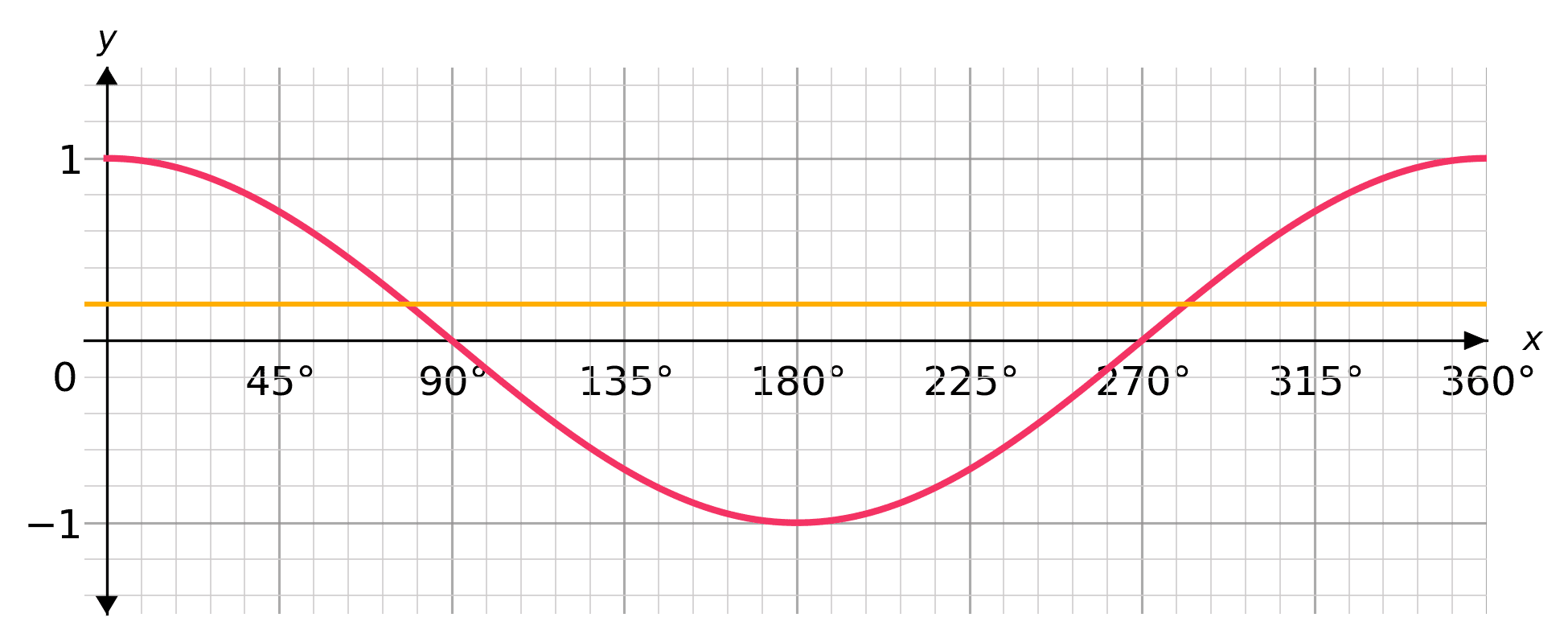The yellow line crosses the cos graph at approximately $x=78\degree$ and $x=282\degree$. These are the approximate solutions to the equation $\cos(x)=0.2$

Tangent graph

To solve, draw a line on the tangent graph at $y=0.2$ and read off the solutions: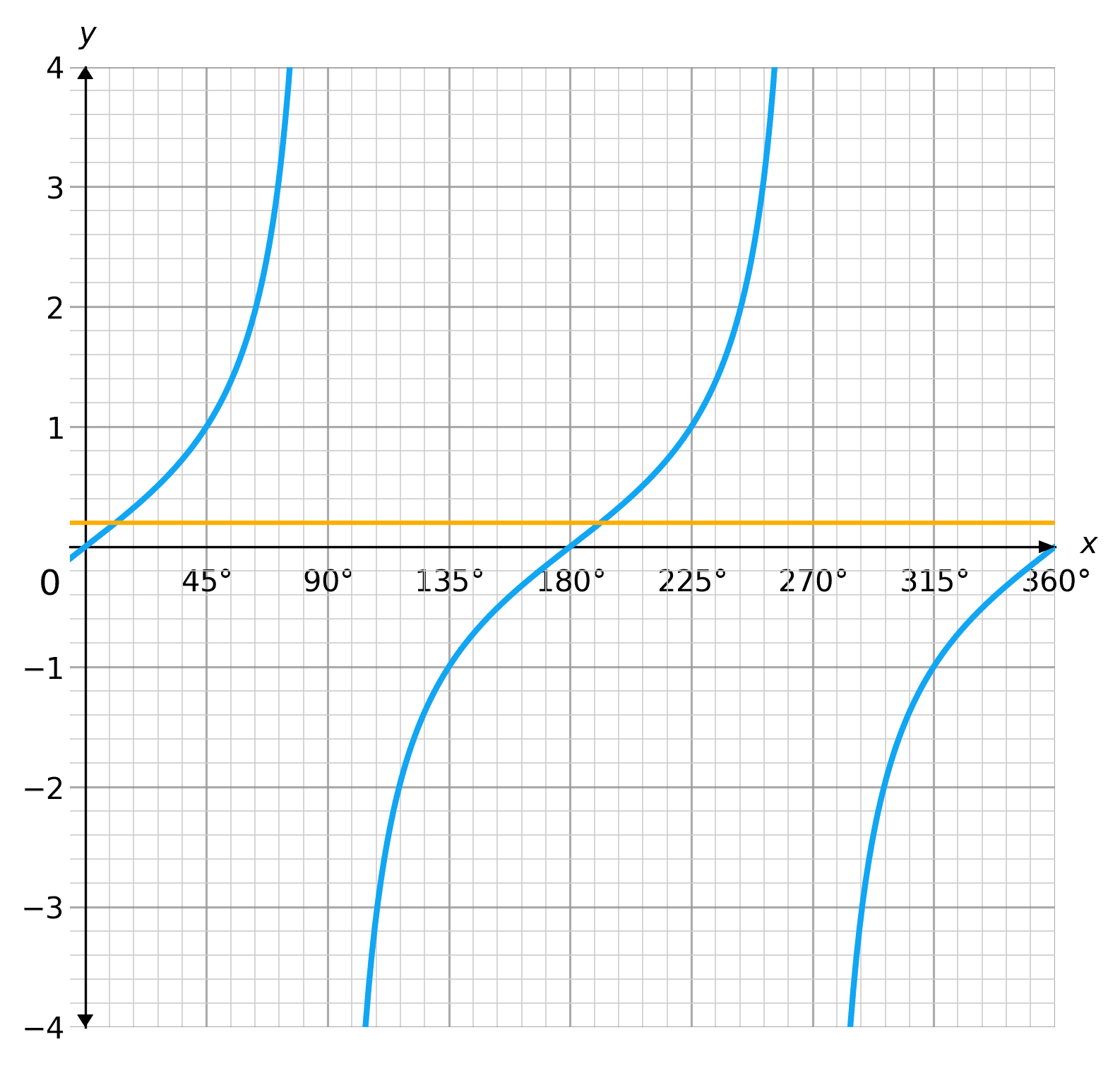The yellow line crosses the tan graph at approximately $x=11\degree$ and $x=191\degree$. These are the approximate solutions to the equation $\tan(x)=0.2$

Level 8-9GCSECambridge iGCSE

## Solving Algebraically – Sine

Sometimes, you may not be given a graph, and so, it would not be possible to accurately solve a trigonometric equation graphically. In this case, we would solve the equation algebraically, using our calculator.

You will always be asked to solve algebraic trigonometric equations for values between $0\degree$ and $360\degree$.

We can use our calculator, and the properties of sine to solve trigonometric equations involving sine.

For example:

$\sin(x)=\dfrac{\sqrt{3}}{2}$

We can use our calculator:

$\sin^{-1}\left(\dfrac{\sqrt{3}}{2}\right)=60\degree$

However, our calculator only gives us one value, but we know there are more solutions between $0\degree$ and $360\degree$.

As the sine graph is positive between $0\degree$ and $180\degree$, we can find the other value by…

$180\degree-60\degree=120\degree$

So the final solutions are $x=60\degree, 120\degree$.

Level 8-9GCSECambridge iGCSE

## Solving Algebraically – Cosine

We can use our calculator, and the properties of cosine to solve trigonometric equations involving cos.

For example:

$\cos(x)=\dfrac{1}{2}$

We can use our calculator:

$\cos^{-1}\left(\dfrac{1}{2}\right)=60\degree$

However, our calculator only gives us one value, but we know there are more solutions between $0\degree$ and $360\degree$.

As the cosine graph is positive between $0\degree$ and $360\degree$, we can find the other value by…

$360\degree-60\degree=300\degree$

So the final solutions are $x=60\degree, 300\degree$.

Level 8-9GCSECambridge iGCSE

## Solving Algebraically – Tangent

We can use our calculator, and the properties of tangent to solve trigonometric equations involving tan.

For example:

$\tan(x)=\sqrt{3}$

We can use our calculator:

$\tan^{-1}(x)=60\degree$

However, our calculator only gives us one value, but we know there are more solutions between $0\degree$ and $360\degree$.

As the tangent graph repeats every $180\degree$, we can find the other value by…

$60\degree+180\degree=240\degree$

So the final solutions are $x=60\degree, 240\degree$.

Level 8-9GCSECambridge iGCSE

## Example 1: Solving Graphically

Use the cosine graph to estimate the solutions to

$\cos(x)=0.4$

between $0\degree$ and $360\degree$

[3 marks]

Draw the line $y=0.4$ onto the graph and read off the solutions: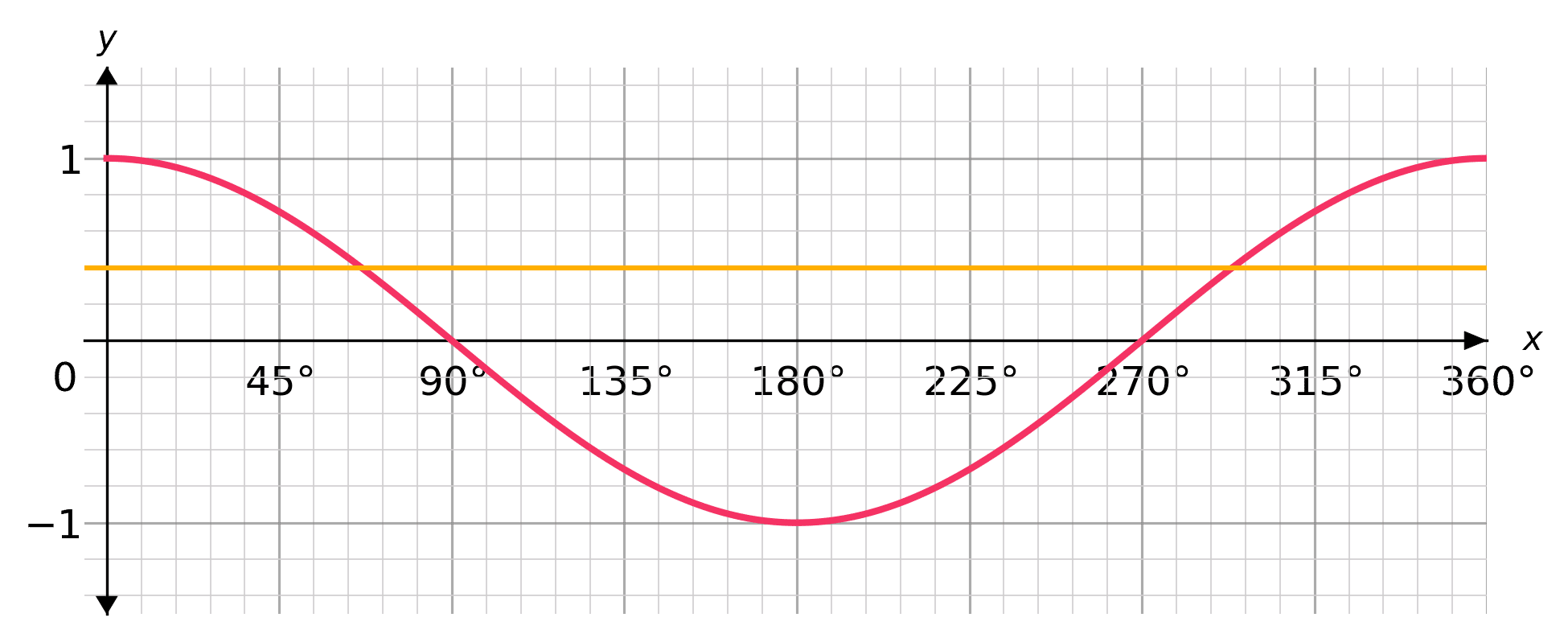The solutions are approximately $x=68\degree$ and $x=293\degree$

Level 8-9GCSECambridge iGCSE

## Example 2: Solving Algebraically

Solve

$\sin(x)=\dfrac{5}{8}$

For values between $0\degree$ and $360\degree$, giving your answers to $2$ decimal places.

[3 marks]

Let’s find the first value using our calculator

$\sin^{-1}\left(\dfrac{5}{8}\right)=38.68\degree$

As sin is positive between $0\degree$ and $180\degree$

$180-38.68=141.32\degree$

Solutions: $x=38.68\degree, 141.32\degree$

Level 8-9GCSECambridge iGCSE

## Trigonometric Equations Example Questions

Draw the line $y=1.3$ and read the solutions: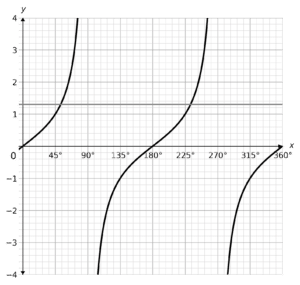Solutions are approximately $x=55\degree\text{, }235\degree$

Using a calculator:

$\tan^{-1}\left(\dfrac{4}{7}\right)=30\degree$

Find the other value:

$30+180=210\degree$

As we are solving for cos, the other value will be

$360-45=315\degree$

We can now do the inverse of $\cos^{-1}(x)$ to work out the value of $x$

$\cos(45)=\dfrac{\sqrt2}{2}$

## You May Also Like...### MME Learning Portal

Online exams, practice questions and revision videos for every GCSE level 9-1 topic! No fees, no trial period, just totally free access to the UK’s best GCSE maths revision platform.

£0.00